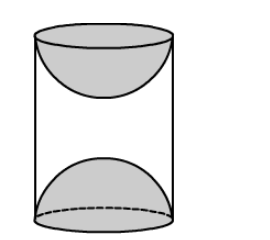# A wooden toy was made by scooping out a hemisphere of same radius from each end of a solid cylinder.`
Question:

A wooden toy was made by scooping out a hemisphere of same radius from each end of a solid cylinder. If the height of the cylinder is 10 cm
and its base is of radius 3.5 cm, then find the volume of wood in the toy.

Solution:We have,

Radius of the cylinder $=$ Radius of the hemispher $=r=3.5 \mathrm{~cm}$ and

Height of the cylinder, $h=10 \mathrm{~cm}$

Now,

Volume of the toy $=$ Volume of the cylinder $-$ Volume of the two hemispheres

$=\pi r^{2} h-2 \times \frac{2}{3} \pi r^{3}$

$=\pi r^{2}\left(h-\frac{4 r}{3}\right)$

$=\frac{22}{7} \times 3.5 \times 3.5 \times\left(10-\frac{4 \times 3.5}{3}\right)$

$=38.5 \times\left(10-\frac{14}{3}\right)$

$=38.5 \times \frac{16}{3}$

$=\frac{616}{3} \mathrm{~cm}^{3}$

$\approx 205.33 \mathrm{~cm}^{3}$

So, the volume of wood in the toy is $\frac{616}{3} \mathrm{~cm}^{3}$ or $205.33 \mathrm{~cm}^{3}$.